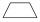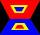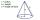# Parallelogram

In the parallelogram we know one internal angle 67°33`. Calculate the other internal angles.

Result

β =  112.45 °
γ =  67.55 °
δ =  112.45 °

#### Solution:Leave us a comment of example and its solution (i.e. if it is still somewhat unclear...):Be the first to comment!## Next similar examples:

1. Isosceles trapeziumTrapezoid YSED (YS||ED) is isosceles. The size of the angle at vertex Y is 17 degrees. Calculate the size of the angle at vertex E.
2. Rectangular trapezoidHow many inner right angles has a rectangular trapezoid?
3. Rhombus anglesIf one angle in the rhombus is 159°, what is it neighboring angle in rhombus?
4. Angles 1It is true neighboring angles have not common arm?
5. Obtuse angleWhich obtuse angle is creating clocks at 17:00?
6. DisjointHow many elements have union and intersection of two disjoint sets when the first have 1 and secodn 8 elements.
7. Street numbersLada came to aunt. On the way he noticed that the houses on the left side of the street have odd numbers on the right side and even numbers. The street where he lives aunt, there are 5 houses with an even number, which contains at least one digit number 6.
8. Cupcakes 2Susi has 25 cupcakes. She gives 4/5. How much does she have left?
9. RoundingThe following numbers round to the thousandth:
10. WithdrawalIf I withdrew 2/5 of my total savings and spent 7/10 of that amount. What fraction do I have in left in my savings?
11. Simple equationSolve the following simple equation: 2. (4x + 3) = 2-5. (1-x)
12. Negative in equation2x + 3 + 7x = – 24, what is the value of x?
13. TeacherTeacher Rem bought 360 pieces of cupcakes for the outreach program of their school. 5/9 of the cupcakes were chocolate flavor and 1/4 wete pandan flavor and the rest were vanilla flavor. How much more chocolate flavor cupcakes than vanilla flavor?
14. Pizza 4Marcus ate half pizza on monday night. He than ate one third of the remaining pizza on Tuesday. Which of the following expressions show how much pizza marcus ate in total?
15. AlleyAlley measured a meters. At the beginning and end are planted poplar. How many we must plant poplars to get the distance between the poplars 15 meters?
16. The diagramThe diagram is a cone of radius 8cm and height 10cm. The diameter of the base is. ..
17. Equation 29Solve next equation: 2 ( 2x + 3 ) = 8 ( 1 - x) -5 ( x -2 )